Trial ends in

# Optimizing Brillouin Optical Time-Domain Analyzers Based on Gain Spectrum Engineering

doi: 10.3791/61115 Published: May 11, 2020
Cheng Feng, Thomas Schneider

### Abstract

Demonstrated is a unique method for sensing performance enhancement in Brillouin optical time-domain analyzers (BOTDA). A Brillouin gain spectrum (BGS) is superimposed with two symmetric Brillouin loss spectra (BLS). This leads to a complex engineered spectrum shape that is more resistant to the sensing system noise. Instead of only one pump and probe interaction as in the conventional BOTDA setup, three optical probe waves are exploited, with one probe located in the BGS and the other two symmetrically in the BLS. Due to the resistance and insensitivity of the engineered spectrum shape to the noise, the sensing performance is enhanced by 60% and the measurand resolution is doubled.

### Introduction

Distributed fiber sensing (DFS) is a unique mechanism that employs a whole fiber as a sensing medium. It has attracted a lot of interest due to the low fiber loss; small size; and the ability to be easily embedded in various structures, such as dams, bridges, and buildings, to perform environment surveillance as an artificial nerve system. In comparison to applying numerous traditional point sensors, such as fiber Bragg gratings (FBG), it provides a more efficient and cost-effective solution in a wide range of large-scale sensing tasks, such as infrastructure and structural health monitoring1.

Current distributed sensors exploit different scattering mechanisms inside the fiber to measure temperature and strain distribution. Among them, DFS based on Brillouin scattering is the most attractive due to the striking advantages of the stimulated Brillouin scattering (SBS), such as high signal-to-noise ratio (SNR), low threshold, and the sensitivity to both temperature2 and strain3. SBS can be classically described as an interaction between the incident optical continuous waves (CW), i.e., the pump, and the counter-propagating CW probe wave via an acoustic wave. According to the conservation of energy and momentum, the probe wave is frequency downshifted to the pump. This shift is called Brillouin frequency shift (BFS). Considering the finite lifetime of a 10 ns acoustic wave, there is a finite spectral distribution of the refracted wave, also called Brillouin gain spectrum (BGS), in which the BFS is the frequency difference between the pump wave and the peak center frequency. The interaction between the waves leads to a frequency down-shifted gain region and a frequency up-shifted loss region where the probe wave gets amplified and attenuated, respectively. For a standard single mode fiber (SSMF) in C-Band, the BFS is approximately 11 GHz and the BGS has a Lorentzian shape with an ultra-narrow full-width at half maximum (FWHM) of 10-30 MHz, which can be further reduced to 3.4 MHz with specific techniques4,5,6,7. Based on these characteristics, SBS can also be applied in microwave photonics filters8,9,10, optical filters11, slow and fast light12,13,14, and high resolution optical spectroscopy7,15.

One of the most promising SBS applications is distributed Brillouin sensing. These sensors exploit the BFS dependence on temperature and strain. The first to be demonstrated was the Brillouin optical time-domain analyzer (BOTDA)16, which is the most consolidated time-domain distributed Brillouin sensing technique. It differs from the conventional CW-SBS interaction in that it exploits the SBS interaction between a pulsed pump wave and a probe CW so that the environmental information is locally interrogated on every fiber section. The pump or probe frequency is usually fixed while the probe or the pump frequency is scanned in the vicinity of the BFS. The probe power is recorded for BGS reconstruction and the BFS is ideally the peak frequency of the local BGS at each fiber section. However, due to the inevitable system noise, the BGS peak is usually ambiguous and a fitting algorithm has to be applied17, which leads to a certain estimation error in frequency18 and influences the measurand resolution.

Statistically, the BFS estimation error is inversely proportional to the system signal-to-noise ratio (SNR). The most straightforward way to enhance the SNR is to increase the pump and probe power. However, these are limited by modulation instability (MI)19 and non-local effects (NLE)20,21 to ~20 dBm and -14 dBm, respectively. Numerous techniques, such as coding22 and Raman based inline-amplification23 have been proposed to break these limits. Recently, it has been reported that this frequency error can be minimized by choosing a proper fitting algorithm24. Relatedly, measurements exploiting the Brillouin phase and a linear fitting algorithm are also reported to have a reduced frequency error25, which indicates the potential of a well-engineered BGS to sensing performance enhancement. Another option to enhance SNR is noise reduction. However, according to the traditional point of view, the sensing system noise comes mainly from the detector (i.e., common-mode noise, including dark noise, shot noise, etc.)26,27 and improvement is less likely.

The basic idea of this paper is to engineer the BGS by the superposition of a conventional BGS with two symmetric Brillouin loss spectra (BLS) (see Figure 1). In comparison to a conventional BGS spectrum, which follows a Lorentzian shape, the engineered spectrum is sharper and more robust with the same level of system noise. Thus, the noise has less influence on the determination of the peak frequency. This can be verified by collecting and fitting the BGS measurement data a statistically significant number of times. Because of this better resistance to the noise, sensing performance enhancements are achieved, including the sensing range by 60% and doubled measurand resolution, i.e., a 50% reduced frequency error. Due to the involvement of the measurement with Brillouin loss interaction in part of the engineered BGS, a direct comparison of the trace noise with and without Brillouin interaction is made. Owing to the circumvention of the excess Brillouin noise, the trace with the engineered BGS is much clearer.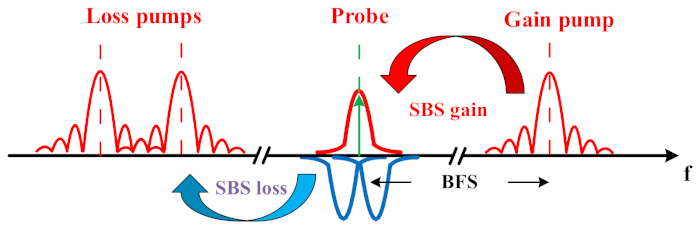Figure 1: Schematic of an engineered BGS by the superposition of one Brillouin gain and two symmetric Brillouin loss spectra. Please click here to view a larger version of this figure.

### Protocol

1. Selecting optimized parameters for the spectrum engineering via simulation

1. Model the engineered BGS gSBS(ν,z) with the equations28,29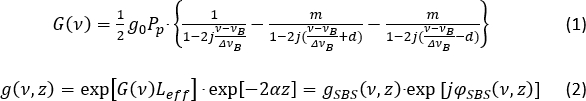as implemented by, for example, the supplemental MATLAB script.
NOTE: Here, G(ν) is the complex gain coefficient, calculated in the script as G_complex within the SBS_g function for the conventional BGS or SBS_gl function for the engineered BGS; g(ν,z) is the local complex Brillouin gain; and gSBS and φSBS are the real and imaginary part of g(ν,z) and symbolize the BGS and SBS phase response at the location z from the pump launching end, respectively. In the script, gSBS is symbolized by the variables SBS_g_log and SBS_gl_log for the conventional and engineered BGS in logarithmic scale, respectively. Pp = 20 dBm (P_pump in the script) is the pump pulse peak power at the fiber launching end; νB is the BFS (normalized in the script); g0 = 0.2 W-1m-1 (g0 in the script) is the Brillouin gain coefficient; ΔνB = 50 MHz (gamma_B in the script) is the FWHM of the BGS; α = 0.2 dB/km is the fiber loss coefficient and is represented by alpha_log and alpha_lin as the value in logarithmic and linear scale in the script, respectively; ν is the probe wave frequency so that the pump and probe frequency offset ν - νB is scanned from -250 MHz to 250 MHz. The pump and probe frequency offset is represented in the script as the global vector f. The term g1 = mg0 is the loss factor; 2d∙ΔνB is the frequency offset between the two losses; and Leff = 10 m (L_eff in the script) is the Brillouin interaction length for 100 ns pulse width. The engineered spectral shape can be easily adjusted by tuning two normalized factors, m and d. The conventional BGS can be modelled simply by m = 0.
2. Add random noise (i.e., additive white Gaussian noise) with the same level both on the conventional and engineered BGS. Adjusting the parameter noise_level in the script can change the noise level.
3. Fit the conventional noisy BGS with the Lorentzian function (Lorentz_g_gain_fun function in the script) and the engineered noisy BGS with the superimposed Lorentzian function (Lorentz_gl_gain_fun function in the script) (see Figure 2A).
4. Determine the peak frequency offset due to the noise for the conventional and engineered BGS (Figure 2B).
5. Repeat steps 1.2-1.4 for N = 500 and collect all the peak frequency offsets Δfci and Δfpi (represented by delta_g_g and delta_g_gl in the script, respectively) for the conventional and engineered BGS in the ith process, respectively.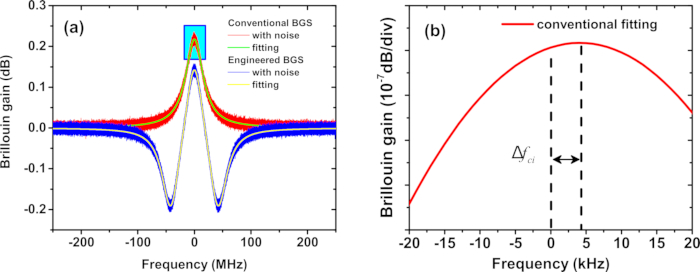Figure 2: Simulated BGS. (A) Demonstration of the fitting of a typical Lorentzian (red) and engineered (blue) BGS in the simulation. (B) The Lorentzian BGS peak from (A). Δfci represents the BFS estimation error for the conventional BGS in the ith measurement. Please click here to view a larger version of this figure.

1. Calculate the ratio of the standard deviation of the noise-induced BFS estimation errors as: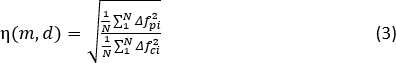2. Plot η as a function of m and d (see Figure 3A). Find the minimum value ηmin and the corresponding m and d. The advantage of the estimation accuracy will be maintained along the whole fiber28.
3. Tune the value of z (i.e., the fiber length, also z in the script) in Equation (2) from 0 km to 60 km, step 50 m and repeat steps 1.1-1.7 with some selected m and d values. Then the ratio of the standard deviation as a function of the fiber length can be acquired (see Figure 3B).

2. Prepare and test the conventional BOTDA setup (highlighted block in Figure 4B)

1. Turn the laser diode (LD) on and check the functionality of the LD with the optical spectrum analyzer (OSA). Usually, a wavelength around 1,550 nm is used.
2. Connect the LD with the 10:90 optical coupler (OC). Check the power from the 10% OC output. If the power is higher than 13 dBm (the maximum input power of the polarization scrambler [Pol.S.]), lower the LD output power by lowering the LD current.
3. Connect the 10% OC output with the Pol.S. In order to avoid polarization fading1, set the scrambling frequency to 1 kHz.
4. Check the power from the output of the Pol.S. If the power is higher than -3 dBm (the maximum input power limit of the semiconductor optical amplifier [SOA]), add attenuators until the power requirement is satisfied. Connect the Pol.S. with the SOA.
5. Apply the electrical pulse train signal with 100 ns pulse width and 4 kHz repetition rate from the pulse generator (PG) on the SOA. Ensure that the amplitude of the pulse signal is higher than the transistor-transistor logic (TTL) threshold of the SOA (i.e., 4 dBm) and the repetition rate satisfies the round-trip requirement30.
6. Connect the output from the SOA with the Erbium-doped fiber amplifier (EDFA 1) and operate the EDFA in automatic current control (ACC) mode. Connect the output from EDFA 1 to port 1 of the circulator (Cir).
7. Check the optical pulse signal from port 2 of Cir in the digitizer by connecting it with the photodiode (PD). In order to avoid any damage to the PD, sufficient attenuation is necessary.
8. Calculate the optical pulse peak power by the waveform measured in the digitizer. The optical peak power Po is estimated according to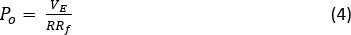where VE is the electrical pulse peak voltage, and R and Rf are the PD responsivity and transresistance. Take the protection attenuation into account in this calculation so that a correct input pump power into the fiber under test (FUT, 10.6 km) is evaluated.
9. Note the EDFA current value I20 when the calculated optical pulse peak power reaches 20 dBm (MI threshold19). The extinction ratio (ER) of the optical pulse train can be evaluated according to the conservation of energy: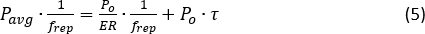where Pavg is the optical average power of the pulse train; frep is the repetition rate; and τ is the pulse width. The ER of the SOA is usually more than 30 dBm, efficiently avoiding NLE31,32.
NOTE: Switch off the EDFA before disconnecting the PD and continuing to build the system.
10. Connect port 2 of the Cir with one end of the FUT to complete setting up the pump branch. Check if the system will suffer from MI by checking the spectrum from the other end of the FUT in the OSA and setting the EDFA at a constant current value, I20. If the detected spectrum is the same (in shape, not necessarily in amplitude) to the one measured in step 2.1, then the system is free from MI. Otherwise, when obvious spectrum broadening or peak splitting33 are observed, reduce the EDFA 1 current to maintain the spectrum shape.
11. Connect the 90% output of the OC to a 50:50 OC 1 and connect one of the OC 1 outputs to the Mach-Zehnder modulator (MZM 1) via a polarization controller (PC). Set the polarization correctly, so that the output from MZM 1 is maximized (polarization alignment).
12. Apply the radio frequency (RF) signal from the RF generator (RFG 1) with the BFS of the FUT (for SSMF around 11 GHz) and 16 dBm amplitude to the MZM 1. Set the DC bias voltage of the MZM 1 so that the carrier is suppressed to the minimum.
13. Connect the output of the MZM 1 with fiber Bragg grating (FBG 1). Check the output spectrum from FBG 1 in the OSA so that the FBG 1 is set to block the upper frequency sideband and the carrier.
14. Connect the output from the FBG 1 to the EDFA 2, another 50:50 OC 2, and an isolator (ISO) consecutively.
15. Operate EDFA 2 also in ACC mode and set the current value to Ig, so that the output power from the ISO is lower than -14 dBm, minimizing NLE20. Switch off the EDFA before disconnecting the power meter and continue the system building. Connect the ISO output to the other end of the FUT to complete the setup building of the gain probe branch.
16. Connect the Cir port 3 to the EDFA 4 (for pre-amplification34) and PD. Set the EDFA to ACC mode. The current value I4 should let the output power from EDFA be 4 smaller than the input limit of the PD. Connect the PD RF output to a 10 MHz RF low pass filter35,36. Connect the filter output to the digitizer.
17. Connect the trigger of the digitizer with the synchronized (or inverse) output of the pulse generator. Set the digitizer parameters as follows: Sampling rate: 500 MSa/s; averaging: 4,096 times; number of samples: 10,000.
18. Set the current value of EDFA 1, EDFA 2, and EDFA 4 to I20, Ig, and I4, respectively. Run a trace recording program. Check the trace measured in the digitizer. If the trace amplitude follows the exponential decay, then the sensing system is MI free. Otherwise, reduce the current value of EDFA 1.

3. Measurement using the conventional BOTDA setup and data processing

1. Set the values of EDFA 1 and EDFA 2 to I20 and Ig, respectively. Scan the frequency of RFG 1 in the range of BFS ± 90 MHz with 1 MHz steps. Record the trace from the recording program after each scanning step.
2. Calculate the local Brillouin gain by dividing the trace amplitude (in Brillouin interaction period) by each DC offset (non-Brillouin interaction period).
3. Retrieve the BGS at each fiber section by fitting the measured noisy BGS with the Lorentzian fitting. Determine the FWHM of the conventional BGS ΔνB0 from the fitting.
4. Repeat steps 3.1 and 3.2 for N = 48 times and collect all the estimated peak (BFS) frequency distributions along the fiber νBci(z) in the ith process (see Figure 5A).
5. Calculate the BFS estimation error as the standard deviation of the fitted BFS at each fiber section in the 48 measurements (see Figure 5B).

4. Preparing the rest of the setup

NOTE: In this case, m = 1 and d = 1.24 were used, per simulation results (see section 1 and Figure 3).

1. Connect the other output of the 50:50 OC 1 in the probe branch with the EDFA 3 and the 50:50 OC 3.
2. Connect one of the outputs of 50:50 OC 3 to a PC and MZM 2. Adjust the polarization so that the output from MZM 2 is maximized.
3. Apply the RF signal from RFG 2 with BFS - d∙ΔνB0 and 16 dBm amplitude on MZM 2. Check the modulation spectrum in the OSA and adjust the bias voltage to minimize the carrier amplitude.
4. Connect the output from MZM 2 to the input of an optical switch (OS 1), one of the inputs of 50:50 OC 4, and the FBG 2.
5. Check the optical signal in the OSA and adjust the FBG 2 center wavelength so that the carrier and the lower frequency sideband are blocked (see Figure 4A). The setup of the loss 1 probe branch will then be complete.
6. Connect the other output of the 50:50 OC 3 to a PC and MZM 3. Adjust the polarization so that the output from MZM 3 is maximized.
7. Apply the RF signal from the RFG 3 with BFS + d∙ΔνB0 and 16 dBm amplitude on MZM 3. Check the modulation spectrum in the OSA and adjust the bias voltage to minimize the carrier amplitude.
8. Connect the output from MZM 3 to a variable optical attenuator (VOA) with a switch function (OS 2) and the other input of 50:50 OC 4. Considering that the offset between the loss probe frequencies are relatively small in comparison to the FBG 2 transmission window (several GHz), the carrier and the lower frequency sideband from MZM 3 will also be blocked by FBG 2. The setup of the loss 2 probe branch will then be complete.
9. Close the OS 1, open the OS 2, and set the EDFA 3 current value to Il so that the power of the loss 1 probe equals -14 dBm (m = 1).
10. Open the OS 1, close the OS 2, and adjust the attenuation of VOA so that the power of the loss 2 probe also equals -14 dBm.

5. Measurement using the complete proposed BOTDA setup and data processing

1. Close the OS 1, close the OS 2, and set the current value of EDFA 1, EDFA 2, EDFA 3, and EDFA 4 to I20, Ig, Il, and I4, respectively. Scan the frequency of the RFG 1 in the range of BFS ± 90 MHz in 1 MHz steps. Frequencies from RFG 2 and RFG 3 scan correspondingly. Record the trace from the program after each scanning step.
2. Calculate the local Brillouin gain as in step 3.2 and retrieve the engineered BGS at each fiber section by fitting the measured noisy BGS with the superimposed Lorentzian function.
3. Repeat steps 5.1 and 5.2 for N = 48 times and collect all the estimated peak (BFS) frequency distributions along the fiber νBpi(z) in the ith process (see Figure 5A).
4. Calculate the BFS estimation error as the standard deviation of the fitted BFS at each fiber section in the 48 measurements (see Figure 5B).

### Representative Results

Figure 3 shows the simulation results. Points with η < 1 in Figure 3A indicate a smaller frequency error (higher measurand resolution) with the engineered BGS. The lower the value was, the bigger the advantage. The minimum ratio was at m = 1, indicating that a multiprobe instead of multipump scheme can be carried out (see Discussion). Figure 3B shows the distribution of the frequency error ratio η along the fiber with the selected parameters for m and d. The dash line indicates the sensing range extension under the same frequency error tolerance (measurand resolution requirement). The maximum sensing range extension of 60% is shown. The experiment was conducted with the setup scheme illustrated in Figure 4B. Figure 6A shows the typical conventional and proposed BGS with their corresponding fittings. The time domain traces of the selected points on the spectrum are depicted in Figure 6B. The BFS in both methods were determined from the peak frequencies of the fittings. The BFS distribution along the fiber examined by both methods is plotted in Figure 5A. After 48 measurements, the standard deviations of the 48 determined BFS at each fiber section were calculated and plotted in Figure 5B. Due to the exponential decreasing SNR, the frequency errors also follow an exponential increase.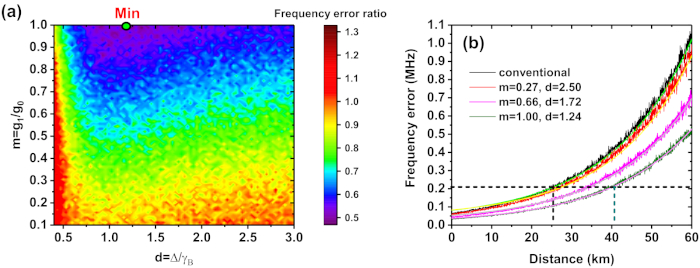Figure 3: Simulation results. (A) The frequency error ratio as a function of m and d in the simulation after 500 calculations. The minimum ratio of 47% was achieved with m = 1 and d = 1.24 (highlighted point). (B) The distribution of the frequency error ratio along the fiber with selected m and d values. The dashed lines indicate the sensing range extension under the same frequency error tolerance. Please click here to view a larger version of this figure.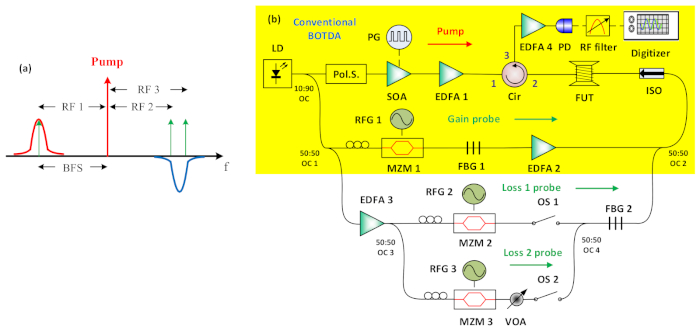Figure 4: Experimental setup scheme. (A) The experimental multiprobe scheme and (B) the setup in detail. The highlighted part of the setup denotes a conventional BOTDA system. LD: laser diode, OC: optical coupler, Pol.S.: polarization scrambler, PG: pulse generator, SOA: semiconductor optical amplifier, EDFA: Erbium-doped fiber amplifier, Cir: circulator, RFG: radio frequency generator, MZM: Mach-Zehnder modulator, FBG: fiber Bragg grating, ISO: isolator, OS: optical switch, VOA: variable optical attenuator, PD: photodiode, FUT: fiber under test. Please click here to view a larger version of this figure.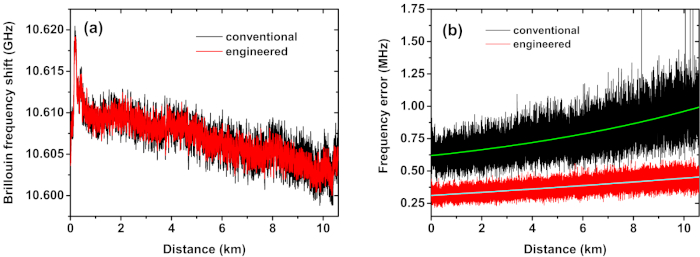Figure 5: Experimental results. (A) BFS distribution along the fiber with the conventional (black) and engineered (red) BGS28. The estimated BFS with the engineered BGS showed the same but clearer distribution along the fiber compared to the conventional BGS, which indicated the better functionality of the proposed sensor. (B) The frequency error distribution along the fiber with conventional (black) and engineered (red) BGS and their corresponding exponential fittings (highlighted)28. The standard deviation verified that the frequency error was reduced by a factor of two. Thus, under the same frequency error tolerance, the sensing range could be drastically extended. Please click here to view a larger version of this figure.Figure 6: Experimental results. (A) The conventional (black) and engineered (red) BGS with their corresponding fittings28. (B) The time domain traces at the maximum gain of the conventional (black) and engineered BGS (blue), and at the maximum loss of the engineered BGS (orange), corresponding to the points A, B, and C in the frequency domain labelled in (A), respectively. The different noise amplitude between the traces indicated that the sensing system suffered not only from the common-mode noise but was also significantly influenced by an active noise source from the SBS interaction. This Brillouin intensity noise level was highly pump power dependent (comparison between trace A and B) and can be avoided in Brillouin loss interactions (trace C). Please click here to view a larger version of this figure.

### Discussion

The most critical step during the experiment is the equalization of the three probe powers so that m = 1 and symmetry between the two Brillouin loss spectra is achieved. Besides the separate power check using the power meter at Cir port 2, as presented in steps 4.9 and 4.10, the power equalization can be more precisely checked in the digitizer. By setting the RF 1 frequency to ~11 GHz (the fiber BFS) and switching off EDFA 3, the conventional trace with the peak gain can be recorded in the digitizer (trace I). Then the RF 2 and RF 3 frequency are set to BFS - d∙ΔνB0 and BFS + d∙ΔνB0, respectively. By switching EDFA 2 off but EDFA 3 on, the trace with the maximum loss in the lower (trace 2) and upper frequency (trace 3) are recorded by closing OS 1 and OS 2, respectively. When all three traces have the same amplitude as the DC offset (SBS gain is equal to the loss), the three probe powers are well equalized. Fine tuning the current value of EDFA 2, EDFA 3, and the attenuation of VOA can adjust the amplitude of the three traces individually.

The implementation of the RF filter before the PD not only reduces the common-mode noise level35,36, but also avoids four wave mixing (FWM) and interference between the loss probe waves. Therefore, the optimal filter bandwidth is not only pump pulse width dependent35, but also dependent on the parameter d. A suitable RF filter should effectively block the beating frequency components between the loss probe waves, which would be in the range of several hundred MHz. This can be checked by the RF spectrum in an electrical spectrum analyzer after the PD, when both loss probe waves are launched into the fiber.

The implementation of the multiprobe scheme restricts the constant m to m = 1. However, this is not a disadvantage, because according to the simulation results, the best performance will be achieved with m = 1. Also, the multiprobe scheme not only simplifies the experimental setup, but also circumvents FWM caused by the copropagation of multipulses.

The significance of the protocol is the enhancement of the SNR in an unprecedented, novel way. The well-engineered spectrum sharpens the peak and reduces the ambiguity against the system noise. Though this paper only demonstrates an instance where 100 ns pulses (10 m spatial resolution) are applied, this method is still valid when the pulse width is shortened and the FWHM of the generated conventional BGS is broadened due to the finite phonon lifetime. The only difference is that the optimal m and d values may vary. Theoretically, a BGS in a delta-function profile will remove all the ambiguity. However, it is hard to achieve this limit due to the finite lifetime of the acoustic wave. This work will inspire new investigations on further optimization of the gain spectrum with a higher contrast. Sensors using the proposed technique will be very valuable to sensing tasks requiring long sensing ranges or high measurement accuracy.

### Disclosures

The authors declare that they have no competing financial interests. Thomas Schneider is an employee of Technische Universität Braunschweig. Cheng Feng receives funding from German Research Foundation and Niedersächsisches Vorab.

### Acknowledgments

Cheng Feng wishes to acknowledge the financial support from German Research Foundation (SCHN 716/13-1, 716/15-2, 716/18-1, 716/26-1) and Niedersächsisches Vorab (NL-4 Project "QUANOMET").

### Materials

 Name Company Catalog Number Comments Current controller for laser diode ILX Lightwave LDX3220 Digitizer Acqiris SA U5309A-1039 Erbium doped fiber amplifier 1 Photop PTEDFA-A-PA-C-SCH-15 Erbium doped fiber amplifier 2 LiComm OFA-TCH Erbium doped fiber amplifier 3 Calmar Optcom AMP-ST30 Erbium doped fiber amplifier 4 Photop PTEDFA-A-PA-C-SCH-15 Fiber Bragg grating 1 Advanced Optics Solutions T-FBG Fiber Bragg grating 2 Advanced Optics Solutions T-FBG Fiber under test ofs Isolator General Photonics S-15-NTSS Laser diode 3SP Group A1905 LMI Mach-Zehnder modulator 1 Avanex IM10 Mach-Zehnder modulator 2 Avanex IM10 Mach-Zehnder modulator 3 Avanex IM10 Nanosecond driving board for semiconductor optical amplifier Highland Technology T160-9 (28A160-9C) Optical coupler 10:90 Newport Benchtop coupler/WDM Optical coupler 50:50 Newport Benchtop coupler/WDM Optical spectrum analyzer Hewlett Packard 86145A Optical switch 1 JDSU SN12-1075NC Photodiode Thorlabs D400FC Polarization scrambler General Photonics PSY-101 Pulase generator Hewlett Packard 8082A Radio function generator 1 Anritsu MG3692C Radio function generator 2 Agilent Technology E8257D Radio function generator 3 HTM T2100 Semiconductor optical amplifier Thorlabs SOA1013SXS Temperature controller for laser diode ILX Lightwave LDT5948 Temperature controller for semiconductor optical amplifier Tektronix TED200 Variable optical attenuator JDSU mVOA-A1 With optical switch function

Video Coming Soon
DOIX